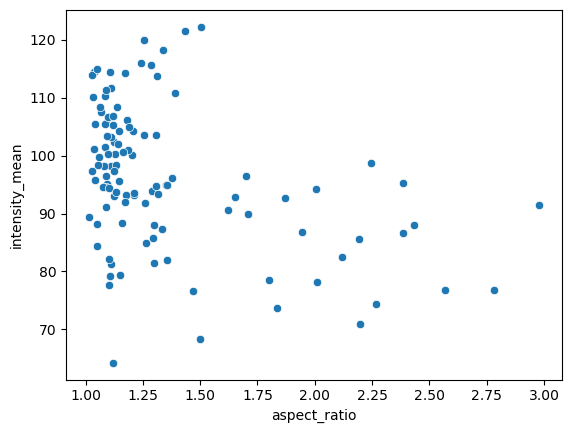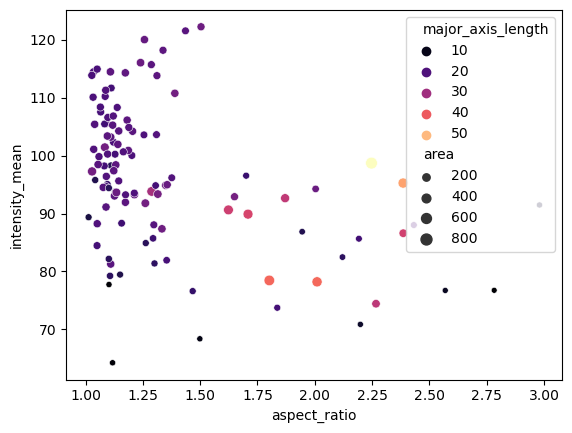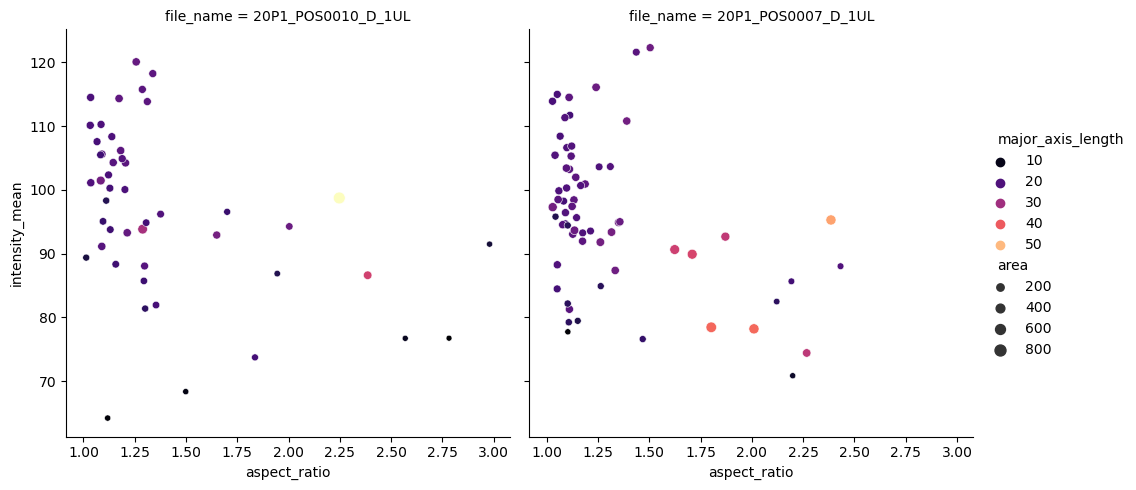# Introduction to Seaborn#

Seaborn is a library for making statistical graphics in Python. It builds on top of matplotlib and integrates closely with pandas data structures.

import seaborn as sns

import numpy as np
import matplotlib.pyplot as plt
import pandas as pd


## Scatter plots with seaborn#

We start by loading a table of measurements into a dataframe df.

df = pd.read_csv("../../data/BBBC007_analysis.csv")
df

area intensity_mean major_axis_length minor_axis_length aspect_ratio file_name
0 139 96.546763 17.504104 10.292770 1.700621 20P1_POS0010_D_1UL
1 360 86.613889 35.746808 14.983124 2.385805 20P1_POS0010_D_1UL
2 43 91.488372 12.967884 4.351573 2.980045 20P1_POS0010_D_1UL
3 140 73.742857 18.940508 10.314404 1.836316 20P1_POS0010_D_1UL
4 144 89.375000 13.639308 13.458532 1.013432 20P1_POS0010_D_1UL
... ... ... ... ... ... ...
106 305 88.252459 20.226532 19.244210 1.051045 20P1_POS0007_D_1UL
107 593 89.905565 36.508370 21.365394 1.708762 20P1_POS0007_D_1UL
108 289 106.851211 20.427809 18.221452 1.121086 20P1_POS0007_D_1UL
109 277 100.664260 20.307965 17.432920 1.164920 20P1_POS0007_D_1UL
110 46 70.869565 11.648895 5.298003 2.198733 20P1_POS0007_D_1UL

111 rows × 6 columns

From this table we can make a scatter plot of aspect ratio versus mean intensity.

sns.scatterplot(data=df, x="aspect_ratio", y="intensity_mean")

<AxesSubplot: xlabel='aspect_ratio', ylabel='intensity_mean'>We can embbed and visualize other features by providing a few extra arguments, for example we can use the area measurement to encode the size of the data points and the major axis length can be used to visualize data points in different colours from the magma color map.

sns.scatterplot(data=df,
x = "aspect_ratio",
y = "intensity_mean",
size = "area",
hue = "major_axis_length",
palette = 'magma')

<AxesSubplot: xlabel='aspect_ratio', ylabel='intensity_mean'>The scatterplot function is an axes-level function. This means, if we want to add subplots, we also need to create a figure and axes from matplotlib first and pass the axes handles.

## Scatter plots with subplots#

Seaborn also has figure-level functions, where the subplots are an argument.

In the example below, we use the relplot function (from relationship) and separate the files by providing ‘file_name’ to the argument col. This allows us to see measurements seprated by the image file the measurements were taken from.

sns.relplot(data=df,
x = "aspect_ratio",
y = "intensity_mean",
size = "area",
hue = "major_axis_length",
col = "file_name",
palette = 'magma')

<seaborn.axisgrid.FacetGrid at 0x148dd2d2be0>